Updated by Joey Johnson on Jun 18, 2014
REPORTJoey Johnson
Owner
11 items   5 followers   0 votes   190 views

# Curated Collection: Solving Word Problems in 1st Grade

Digital Learning Environments Curated Collection: This contains a curated collection of web resources for word problem-solving introduction, strategies, online practice, and games.

1

## Solve a Word Problem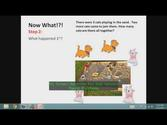Teaches 1st graders how to solve a word problem in 3 easy steps.

2

## Math Island Word Problem Practice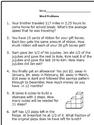This is a group of 5 worksheets with answer sheet to practice on the promethean board with. This contains both addition and subtraction.

3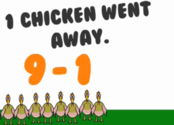4

## Lessons & Instructional Materials | Math Word Problems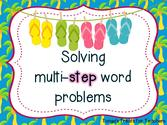Lessons & Instructional Materials - Math Word Problems. The flipchart allows students to identify key words in word problems and includes a sorting activity. Students will drag numbers and math symbols to create addition or subtraction sentences based on word problems..

5

## Subtraction Word Sentences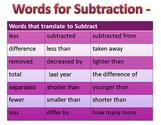Read the word problem and write the correct equation.

6

## Subtraction Word Problems up to 18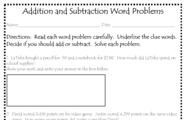Subtraction word problems with your difference being between 0 and 18.

7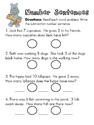Solve addition word problems up to 18.

8

## Numberline Word Problems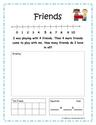Use a number line to solve these word problems.

9

## Word Problem Exercise Game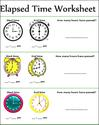An interactive game where you solve the word problem.

10

## Thinking Blocks: Model and Solve Game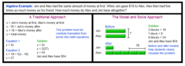Model and Solve word problems with this fun interactive game.

11

## Addition and Subtraction Key Words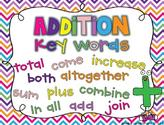Addition and Subtraction Keywords for Students